2402℃

## 概论

• 和HashMap一样，还是通过数组存储元素的

• 图中的黑色箭头表示next指针，这跟HashMap是一样的；
• 图中的红色箭头表示before和after指针，分别指向这个节点的前面和后面一个节点，这就可以实现有序访问；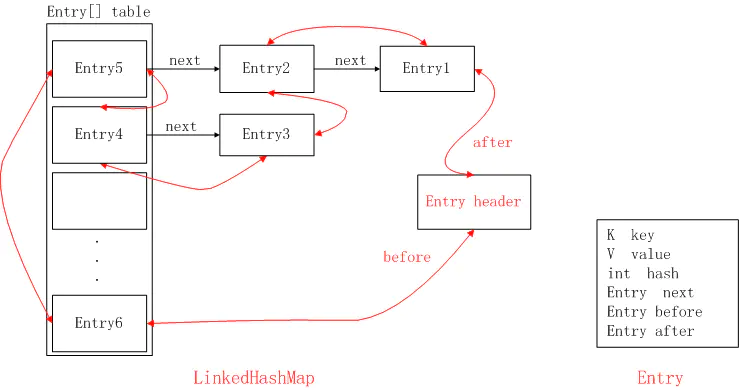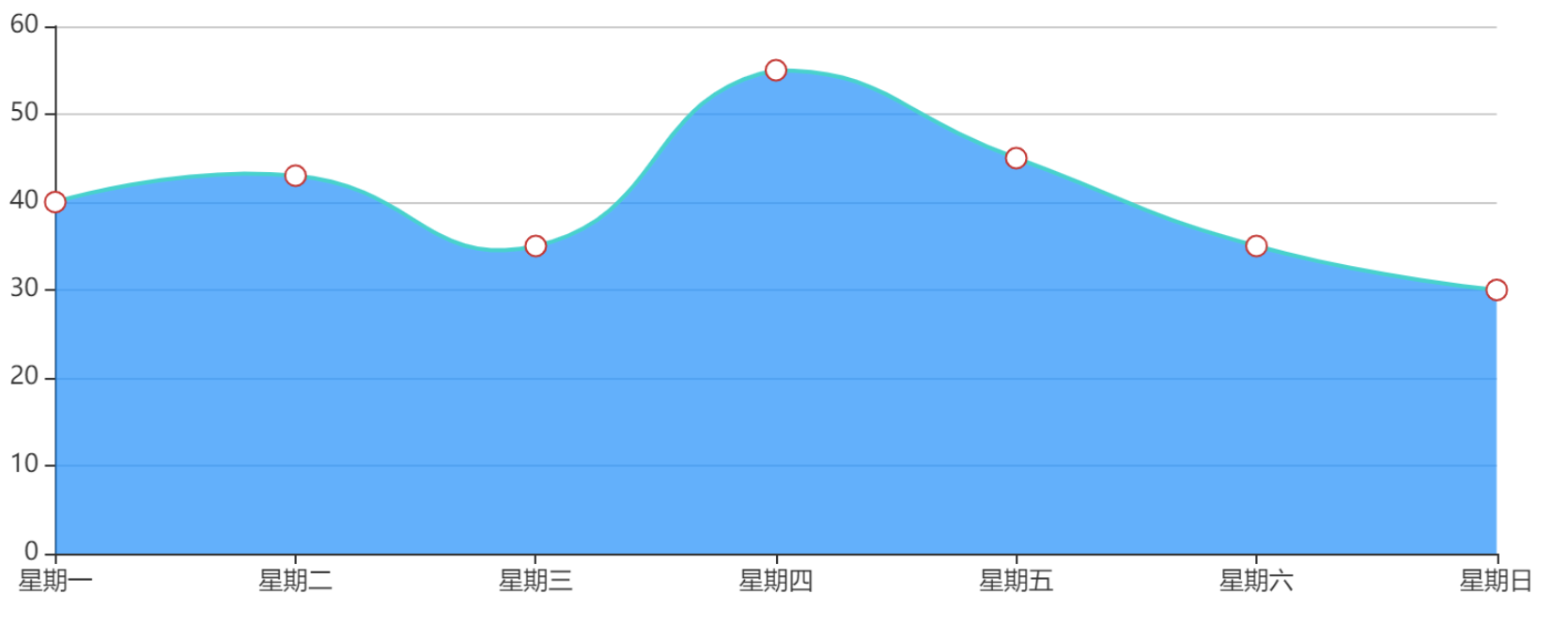HashMap<String, Integer> map = new HashMap<>();
map.put("星期一", 40);
map.put("星期二", 43);
map.put("星期三", 35);
map.put("星期四", 55);
map.put("星期五", 45);
map.put("星期六", 35);
map.put("星期日", 30);
for (Map.Entry<String, Integer> entry : map.entrySet()){
System.out.println("key: " + entry.getKey() + ", value: " + entry.getValue());
}
/**
* 结果如下：
* key: 星期二, value: 40
* key: 星期六, value: 35
* key: 星期三, value: 50
* key: 星期四, value: 55
* key: 星期五, value: 45
* key: 星期日, value: 65
* key: 星期一, value: 30
*/

LinkedHashMap<String, Integer> map = new LinkedHashMap<>();
map.put("星期一", 40);
map.put("星期二", 43);
map.put("星期三", 35);
map.put("星期四", 55);
map.put("星期五", 45);
map.put("星期六", 35);
map.put("星期日", 30);
for (Map.Entry<String, Integer> entry : map.entrySet()){
System.out.println("key: " + entry.getKey() + ", value: " + entry.getValue());
}

key: 星期一, value: 40
key: 星期二, value: 43
key: 星期三, value: 35
key: 星期四, value: 55
key: 星期五, value: 45
key: 星期六, value: 35
key: 星期日, value: 30

### 继承关系图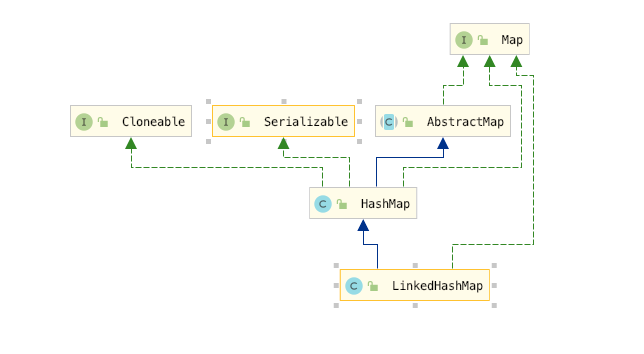### 构造方法

// 构造方法1，构造一个指定初始容量和负载因子的、按照插入顺序的LinkedList
accessOrder = false;
}
super(initialCapacity);
accessOrder = false;
}
super();
accessOrder = false;
}
public LinkedHashMap(Map<? extends K, ? extends V> m) {
super(m);
accessOrder = false;
}
this.accessOrder = accessOrder;
}

/**
* The iteration ordering method for this linked hash map: <tt>true</tt>
* for access-order, <tt>false</tt> for insertion-order.
*
* @serial
*/
final boolean accessOrder;

### Entry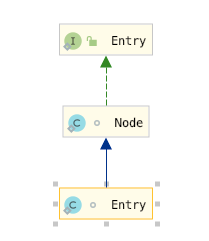static class Entry<K,V> extends HashMap.Node<K,V> {
Entry<K,V> before, after;
Entry(int hash, K key, V value, Node<K,V> next) {
super(hash, key, value, next);
}
}

### accessOrder

/**
* The iteration ordering method for this linked hash map: <tt>true</tt>
* for access-order, <tt>false</tt> for insertion-order.
*
* @serial
*/
final boolean accessOrder;

   /**
*/

/**
* The tail (youngest) of the doubly linked list.
*/
transient LinkedHashMap.Entry<K,V> tail;

### newNode方法

// Create a regular (non-tree) node
Node<K,V> newNode(int hash, K key, V value, Node<K,V> next) {
return new Node<>(hash, key, value, next);
}

Node<K,V> newNode(int hash, K key, V value, Node<K,V> e) {
return p;
}

// link at the end of list 将链表的尾节点和当前节点串起来
// 将tail 的原始值记做last,因为下面要对 tail 赋值
//将新创建的节点p作为尾结点tail
tail = p;
// 如果last是null 也就是当前节点是第一个节点，否则last 不可能是null
if (last == null)
else {
// 此时p是tail节点，那么原来的tail节点将成为 p节点前置节点，p 节点也就是新的节点将成为原来节点的后置节点
p.before = last;
last.after = p;
}
}

### afterNodeAccess 方法

// Callbacks to allow LinkedHashMap post-actions
void afterNodeAccess(Node<K,V> p) { }
void afterNodeInsertion(boolean evict) { }
void afterNodeRemoval(Node<K,V> p) { }

// move node to last 将节点移动到最后(其实从这个注释我们就可以知道，这个方法是干什么的了)
void afterNodeAccess(Node<K,V> e) {
// accessOrder 确定是按照访问顺序的，如果当前节点不是最后节点，因为是的话就不用移了
if (accessOrder && (last = tail) != e) {
//p：当前节点 b：当前节点的前一个节点 a：当前节点的后一个节点；
(LinkedHashMap.Entry<K,V>)e, b = p.before, a = p.after;
//将p.after设置为null，断开了与后一个节点的关系，但还未确定其位置
p.after = null;
// 如果 p 的前置是null 也就是b 是null 那么此时 就是第一个节点也就是head ，因为p 要后移，那么此时p 的后置应该是head 了
if (b == null)
// 如果不是的话，那么p 的后置也就是a 应该代替p 的位置，成为b 的后置  b->p->a  =>  b->a
else
b.after = a;
// 到这里p 已经成为一个游离的节点了，接下来我们只要找到一个位置将p 安置即可，目前的情况是 b->a ，如果a!=null 的话，也让a 指向 b => 也就是 a b 互指  b<->a
if (a != null)
a.before = b;
// 如果a==null 的话，也就是是b 将成为尾部节点，此时第二次完成last 的赋值，此时last 指向b(这里有个问题是e不是tail 此时 a 就不可能是null)
else
last = b;
// 这里就相当于已经找到了尾节点，( 因为上面a!=null,此时last=tail也不是null，所以只会走else )
if (last == null)
else {
p.before = last;
last.after = p;
}
// 完成新的tail 确定，也就是p 节点，这下就知道上面为什么先让 p.after = null 了吧
tail = p;
++modCount;
}
}

final V putVal(int hash, K key, V value, boolean onlyIfAbsent,boolean evict) {
if ((p = tab[i = (n - 1) & hash]) == null)
tab[i] = newNode(hash, key, value, null);
else {
if (e != null) {
// existing mapping for key
V oldValue = e.value;
if (!onlyIfAbsent || oldValue == null)
e.value = value;
// 将存在的节点放到最后(因为存在所以是Access，也就是访问存在的元素)
afterNodeAccess(e);
return oldValue;
}
}
++modCount;
if (++size > threshold)
resize();
// 这里就是插入到了树的叶子节点或者是链表的末尾，也就是说本来就在末尾就不用操作了
afterNodeInsertion(evict);
return null;
}

 @Test
public void test1() {
// 这里是按照访问顺序排序的
HashMap<Integer, Integer> m = new LinkedHashMap<>(10, 0.75f, true);
// 每次调用 put() 函数，往 LinkedHashMap 中添加数据的时候，都会将数据添加到链表的尾部
m.put(3, 11);
m.put(1, 12);
m.put(5, 23);
m.put(2, 22);
// 再次将键值为 3 的数据放入到 LinkedHashMap 的时候，会先查找这个键值是否已经有了，然后，再将已经存在的 (3,11) 删除，并且将新的 (3,26) 放到链表的尾部。
m.put(3, 26);
// 当代码访问到 key 为 5 的数据的时候，我们将被访问到的数据移动到链表的尾部。
m.get(5);
// 那么此时的结果应该是 1 2 3 5 因为 3 和 5 一次被移动到了最后面去
for (Map.Entry e : m.entrySet()) {
System.out.println(e.getKey());
}
}

1
2
3
5

### afterNodeInsertion 方法

 void afterNodeInsertion(boolean evict) {
// possibly remove eldest
if (evict && (first = head) != null && removeEldestEntry(first)) {
K key = first.key;
removeNode(hash(key), key, null, false, true);
}
}

removeEldestEntry 方法的源代码如下

protected boolean removeEldestEntry(Map.Entry<K,V> eldest) {
return false;
}

#### afterNodeInsertion 方法的意义是什么呢

 public V put(K key, V value) {
return putVal(hash(key), key, value, false, true);
}
/**
* @param evict if false, the table is in creation mode.
* @return previous value, or null if none
*/
final V putVal(int hash, K key, V value, boolean onlyIfAbsent,boolean evict) 

public HashMap(Map<? extends K, ? extends V> m) {
putMapEntries(m, false);
}

/**
* Implements Map.putAll and Map constructor.
*
* @param m the map
* @param evict false when initially constructing this map, else
* true (relayed to method afterNodeInsertion).
*/
final void putMapEntries(Map<? extends K, ? extends V> m, boolean evict) {
int s = m.size();
if (s > 0) {
if (table == null) { // pre-size
float ft = ((float)s / loadFactor) + 1.0F;
int t = ((ft < (float)MAXIMUM_CAPACITY) ?
(int)ft : MAXIMUM_CAPACITY);
if (t > threshold)
threshold = tableSizeFor(t);
}
else if (s > threshold)
resize();
for (Map.Entry<? extends K, ? extends V> e : m.entrySet()) {
K key = e.getKey();
V value = e.getValue();
putVal(hash(key), key, value, false, evict);
}
}
}

// Callbacks to allow LinkedHashMap post-actions
void afterNodeAccess(Node<K,V> p) { }
void afterNodeInsertion(boolean evict) { }
void afterNodeRemoval(Node<K,V> p) { }

protected boolean removeEldestEntry(Map.Entry<K,V> eldest) {
return false;
}

public class BuerLinkedHashMap<K, V> extends LinkedHashMap<K, V> {
private int maxCacheSiz;

super();
this.maxCacheSiz = maxCacheSize;
}

@Override
protected boolean removeEldestEntry(Map.Entry<K, V> eldest) {
if (size() > maxCacheSiz) {
System.out.println("即将执行删除");
return true;
} else {
return false;
}
}

public static void main(String[] args) {

for (int i = 0; i < 11; i++) {
}
}
}

即将执行删除

### afterNodeRemoval 方法

void afterNodeRemoval(Node<K,V> e) { // unlink
// 将要删除的节点标记为p， b 是p 的前置，a 是p 的后置
// 切点p 的前后连接
p.before = p.after = null;
// p 的前置是null 的话，p 是要被删除的，那么p 的后置将成为head
if (b == null)
// p 的前置b 不是null 的话，b的前置p指向b的后置a即可， 也就是 b->p->a   => b->a
else
b.after = a;
// a 是null 的话，p 则是原来的tail,那么新的tail 就是 b
if (a == null)
tail = b;
// 如果a 不是null 则是一个普通的节点，也就是说删除的不是tail 则让a 也指向b
else
a.before = b;
}

## debug 源码 插入元素的过程

final V putVal(int hash, K key, V value, boolean onlyIfAbsent,
boolean evict) {
Node<K,V>[] tab; Node<K,V> p; int n, i;
if ((tab = table) == null || (n = tab.length) == 0)
n = (tab = resize()).length;
if ((p = tab[i = (n - 1) & hash]) == null)
// 首先newNode方法是重写了的，在插入元素的同时将元素放到了链表的尾部，具体可以参考上面对这个方法的解析
tab[i] = newNode(hash, key, value, null);
else {
Node<K,V> e; K k;
if (p.hash == hash &&
((k = p.key) == key || (key != null && key.equals(k))))
e = p;
else if (p instanceof TreeNode)
e = ((TreeNode<K,V>)p).putTreeVal(this, tab, hash, key, value);
else {
for (int binCount = 0; ; ++binCount) {
if ((e = p.next) == null) {
p.next = newNode(hash, key, value, null);
if (binCount >= TREEIFY_THRESHOLD - 1) // -1 for 1st
treeifyBin(tab, hash);
break;
}
if (e.hash == hash &&
((k = e.key) == key || (key != null && key.equals(k))))
break;
p = e;
}
}
if (e != null) { // existing mapping for key
V oldValue = e.value;
if (!onlyIfAbsent || oldValue == null)
e.value = value;
// 这里说明这个key 已经存在，就相当于与是对这个key 的一次访问，所以访问结束之后调用了 afterNodeAccess(e)，其实这里还有一点要说明，即使存在的key 是在链表的尾部或者是在树的叶子节点
// 也算是访问，所以这里并没有要求位置，只要求存在，所以afterNodeAccess 中判断了e 是不是 tail
afterNodeAccess(e);
return oldValue;
}
}
++modCount;
if (++size > threshold)
resize();
afterNodeInsertion(evict);
return null;
}

## 获取元素的过程

public V get(Object key) {
Node<K,V> e;
if ((e = getNode(hash(key), key)) == null)
return null;
if (accessOrder)
afterNodeAccess(e);
return e.value;
}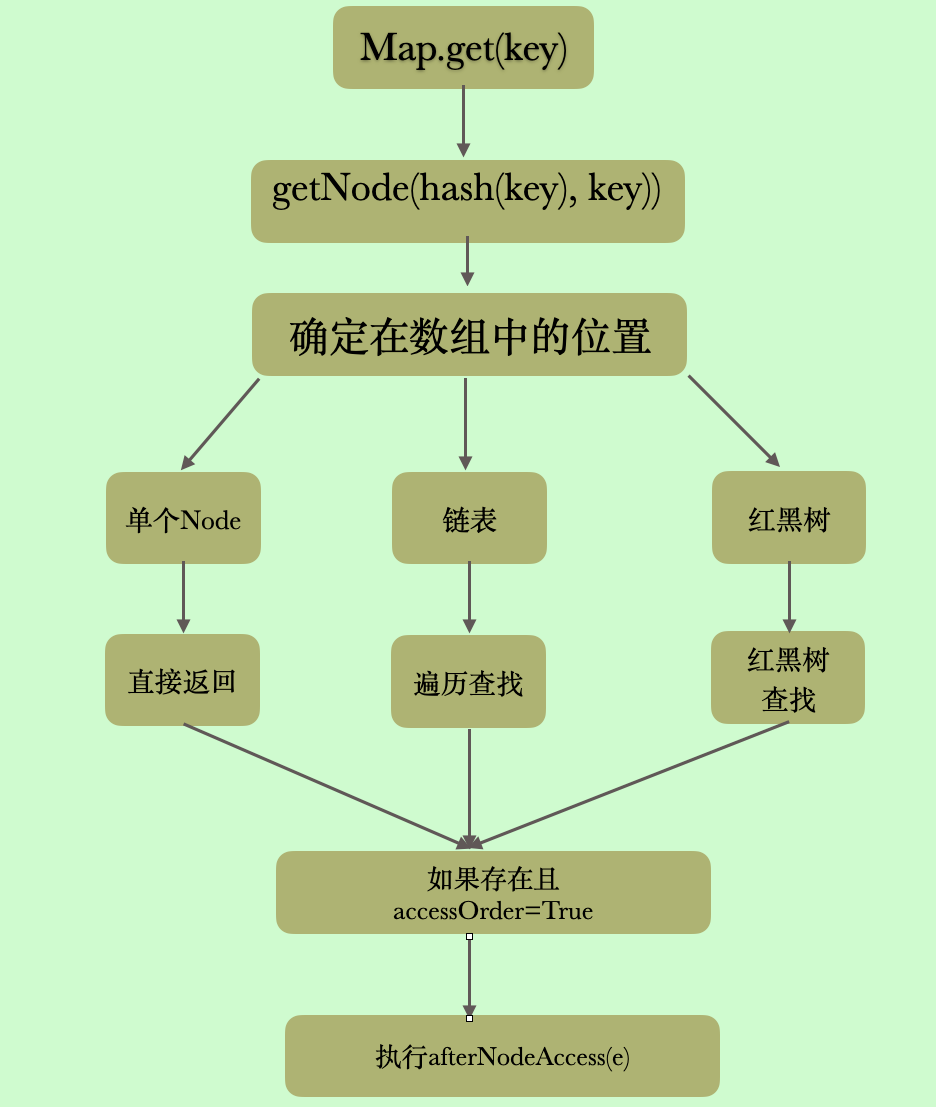## 删除元素的过程

Hashmap 一节，我们也没有讲remove 方法，所以这里我们来看一下，细节的一些东西都写在注释里了，首先remove 方法是有返回值的，存在则返回key 对应的value 不存在则返回null

public V remove(Object key) {
Node<K,V> e;
return (e = removeNode(hash(key), key, null, false, true)) == null ?
null : e.value;
}
// 实际执行删除的方法
final Node<K,V> removeNode(int hash, Object key, Object value,
boolean matchValue, boolean movable) {
Node<K,V>[] tab; Node<K,V> p; int n, index;
// 数组不为空且有元素，并且tab[index = (n - 1) & hash]!=null 也就是这个桶上得有元素，不是空桶 否则返回null
if ((tab = table) != null && (n = tab.length) > 0 && (p = tab[index = (n - 1) & hash]) != null) {
// node 要删除的节点
Node<K,V> node = null, e; K k; V v;
//p 就是当前节点，也就是和我们要删除的key 在同一个桶上的第一个节点，判断p 是不是要删除的节点 是则标记为node
if (p.hash == hash && ((k = p.key) == key || (key != null && key.equals(k))))
node = p;
// 不是的话，开始遍历，可能是链表可能是树，前提是当前节点的next 节点那不为空，如果为空的话，就不用遍历了
//因为当前节点不是要删除的节点，并且当前节点么有next，那就是不存在了
else if ((e = p.next) != null) {
// 判断是不是树
if (p instanceof TreeNode)
node = ((TreeNode<K,V>)p).getTreeNode(hash, key);
else {
// 不是树的话，那就一定是链表了
do {
// 这个判断方式和上面的判断方式是一样的，找到了要删除的节点就跳出循环
if (e.hash == hash &&((k = e.key) == key ||  (key != null && key.equals(k)))) {
node = e;
break;
}
// p 是 e 的前一个节点，当node 是要删除的节点的时候，p 就是待删除节点的前一个节点
p = e;
} while ((e = e.next) != null);
}
}
// 判断有没有找到要删除的节点，并且这里有其他的条件，因为上面判断是不是要删除的节点仅仅判断了key 是不是同一个key
if (node != null && (!matchValue || (v = node.value) == value || (value != null && value.equals(v)))) {
// 树删除
if (node instanceof TreeNode)
((TreeNode<K,V>)node).removeTreeNode(this, tab, movable);
// 此时只有一个节点，也就是也就是一开始的第一个if 处的判断条件为true,就是说要删除节点就是链表的第一个节点
else if (node == p)
tab[index] = node.next;
// 链表删除，此时p 是node 的前一个节点
else
p.next = node.next;
// 标记修改
++modCount;
// 修改size 大小
--size;
afterNodeRemoval(node);
return node;
}
}
return null;
}

matchValue 判断删除的时候，是否要求节点的值是相同的，True 则表示找到key相同的节点之后，还需要判断值是相等的才可以使删除。下面就是判断条件，可以自己分析一下

(!matchValue || (v = node.value) == value || (value != null && value.equals(v)))

## 总结

### 四个维护双向链表的方法

#### afterNodeRemoval(Node<K,V> p)